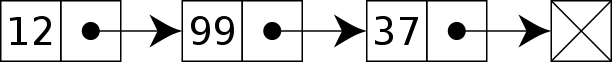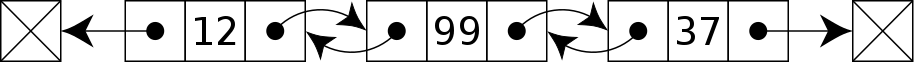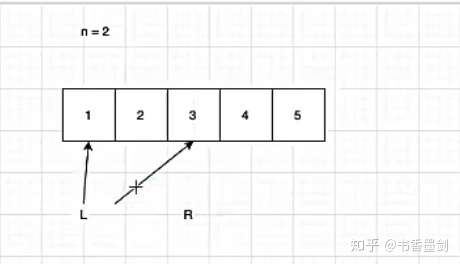# 【青铜三人行】删除链表的倒数第 N 个节点

## 啥是链表``````function ListNode(val) {
this.val = val;
this.next = null;
}``````

## 解法一：两次遍历找到对应的节点

``````/**
* function ListNode(val) {
*     this.val = val;
*     this.next = null;
* }
*/
/**
* @param {number} n
* @return {ListNode}
*/
length = 0;

while (true) {
if (node === null) break;

node = node.next;
length++;
}

if (n === length) return head.next;

for (let i = 0; i < length - n - 1; i++) node = node.next;

node.next = node.next.next;

}``````

## 解法二：转离散节点为连续节点

``````/**
* function ListNode(val) {
*     this.val = val;
*     this.next = null;
* }
*/
/**
* @param {number} n
* @return {ListNode}
*/
const arrNodes = [];

while (true) {
if (node === null) {
const length = arrNodes.length;
else arrNodes[length - n - 1].next = arrNodes[length - n + 1];
break;
}
arrNodes.push(node);
node = node.next;
}
}``````

``if (length === n) head = head.next;``

## 解法三：双指针法``````function removeNthFromEnd(head, n) {
const dummy = new ListNode(0);

let l = dummy,
r = dummy,
offset = n;

while (offset--) r = r.next;

while (r.next) (l = l.next), (r = r.next);

l.next = l.next.next;

return dummy.next;
}``````

## Ertra

``````func removeNthFromEnd(head *ListNode, n int) *ListNode {
i := 0

for p != nil {
if (i > n) {
store = store.Next
}
p = p.Next
i++
}
// 删除头节点
if (i == n) {
return store.Next
}
store.Next = store.Next.Next

}``````